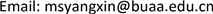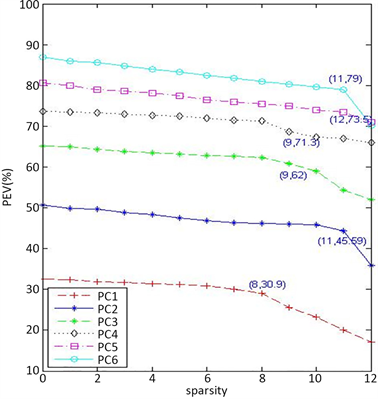﻿ 稀疏主成分分析的两阶段法 Two-Stage Method of Sparse Principal Component Analysis

Vol.06 No.09(2017), Article ID:23167,8 pages
10.12677/AAM.2017.69142

Two-Stage Method of Sparse Principal Component Analysis

Xin Yang

The School of Mathematics and Systems, Beihang University, BeijingReceived: Dec. 1st, 2017; accepted: Dec. 19th, 2017; published: Dec. 26th, 2017ABSTRACT

In this paper, we propose a sparse principal component based on two-stage method, that is, we first get principal component, and then add the ${\mathcal{l}}_{1}$ regular term of the loadings. Coordinate descent method is used to solve the model. The model is easy to understand. In addition, this paper proposes a heuristic algorithm which can determine the penalty parameters in the model. By selecting the appropriate penalty parameters, the sparse principal component explained variance and sparsity can be optimized at the same time.

Keywords:Dimension Reduction, Sparse Principal Component Analysis, Least Absolute Shrinkage and Selection Operator, Coordinate Descent Method1. 引言

2. 稀疏主成分分析的两阶段法

2.1. LASSO问题与SPCA算法

${\beta }^{*}=\underset{\beta \in {ℝ}^{p}}{\mathrm{arg}\mathrm{min}}\frac{1}{2}{‖X\beta -y‖}_{2}^{2}+\lambda {‖\beta ‖}_{1},$

Zou等  2012年提出SPCA模型，SPCA模型先将PCA表述为回归优化问题，然后添加关于回归系

$\begin{array}{l}\underset{A,B}{\text{minimize}}\text{\hspace{0.17em}}\text{\hspace{0.17em}}\text{\hspace{0.17em}}\text{\hspace{0.17em}}\text{\hspace{0.17em}}{‖X-XB{A}^{\text{T}}‖}_{\mathrm{F}}^{2}+\lambda \sum _{j=1}^{k}{‖{\beta }_{j}‖}^{2}+\sum _{j=1}^{k}{\lambda }_{1,j}{‖{\beta }_{j}‖}_{1}\\ \text{subject}\text{\hspace{0.17em}}\text{to}\text{\hspace{0.17em}}\text{}{A}^{\text{T}}A={I}_{k}.\end{array}$ (1)

SPCA利用块坐标下降法将(1)的变量分成 $B$$A$ 两个坐标块，固定其中一个坐标块，求解关于另一个坐标块的子问题，交替求解关于两个变量的子问题直至满足终止条件。SPCA模型将PCA与回归分析建立联系，并且对于不同的数据类型( $n>p$$n\le p$ )都具有较低的计算复杂度  。

SPCA算法初值 ${A}_{0}={B}_{0}$ 均取前 $k$ 个主成分载荷 ${\overline{v}}_{1},\cdots ,{\overline{v}}_{k}$ ，固定 $A$ 求解问题(1)等价于求解

$\underset{B}{\text{minimize}}\text{\hspace{0.17em}}\text{\hspace{0.17em}}\text{\hspace{0.17em}}\text{\hspace{0.17em}}\text{\hspace{0.17em}}{‖XA-XB‖}_{\mathrm{F}}^{2}+\lambda \sum _{j=1}^{k}{‖{\beta }_{j}‖}^{2}+\sum _{j=1}^{k}{\lambda }_{1,j}{‖{\beta }_{j}‖}_{1},$ (2)

${y}_{j}=X{\overline{v}}_{j},j=1,\cdots ,k$ ，求解(2)等价于求解 $k$ 个独立的弹性网问题

${\beta }_{j}^{*}=\underset{{\beta }_{j}}{\mathrm{arg}\mathrm{min}}{‖{y}_{j}-X{\beta }_{j}‖}^{2}+\lambda {‖{\beta }_{j}‖}^{2}+{\lambda }_{1,j}{‖{\beta }_{j}‖}_{1},\text{\hspace{0.17em}}\text{\hspace{0.17em}}j=1,\cdots ,k.$ (3)

2.2. 两阶段法的第一阶段与第二阶段

(1) 样本协方差阵特征值分解

(2) 数据矩阵奇异值分解

$\underset{v\in {ℝ}^{p}}{\text{minimize}}\text{\hspace{0.17em}}\text{\hspace{0.17em}}\frac{1}{2}{‖Xv-{y}_{i}‖}_{2}^{2}+\lambda {‖v‖}_{1},i=1,\cdots ,k,$

$\underset{v\in {ℝ}^{p}}{\text{minimize}}\text{\hspace{0.17em}}\text{\hspace{0.17em}}\frac{1}{2}{‖Xv\text{-}y‖}_{2}^{2}+\lambda {‖v‖}_{1}.$ (4)

2.3. 基于LASSO的坐标下降法

${S}_{\lambda }\left(\alpha \right)=\left\{\begin{array}{l}\alpha -\lambda ,\text{\hspace{0.17em}}\text{\hspace{0.17em}}\alpha >\lambda \\ 0,\text{\hspace{0.17em}}\text{\hspace{0.17em}}|\alpha |\le \lambda \\ \alpha +\lambda ,\text{\hspace{0.17em}}\text{\hspace{0.17em}}\alpha <-\lambda \end{array}$

${v}_{i}^{*}=\underset{v}{\mathrm{arg}\mathrm{min}}\left\{\sum _{j=1}^{n}{x}_{ji}^{2}{v}_{i}^{2}-\left(\sum _{j=1}^{n}{x}_{ji}{r}_{j}^{\left(i\right)}\right){v}_{i}+\lambda |{v}_{i}|\right\},$

${v}_{i}=\frac{{S}_{\lambda }\left({\sum }_{j=1}^{n}{x}_{ji}{r}_{j}^{\left(i\right)}\right)}{\sum _{j=1}^{n}{x}_{ji}^{2}},$ (5)

${v}_{i}=\frac{{S}_{\lambda }\left(〈{x}_{i},y〉-\sum _{m:|{v}_{m}|>0}〈{x}_{i},{x}_{m}〉{v}_{m}+{v}_{i}\right)}{{x}_{i}^{\text{T}}{x}_{i}},$ (6)

2.4. 参数选择算法

LASSO问题中的非负惩罚参数通常使用交叉验证  进行确定，但是这种方法高度依赖于数据矩阵，在已知样本协方差矩阵的数据集中，交叉验证的方法并不适用，因此本文提出一种有效的参数选择方法。稀疏度用 $s$ 表示，下面给出参数选择算法1。

3. 数值结果

3.1. Pitprop数据Figure 1. Six PCs of pitprop data: trade-off curve

3.2. 结肠癌基因数据

3.3. 20新闻组数据Table 1. Six sparse PCs of pitprop data: test index of sparse PCA algorithmsTable 2. Three sparse PCs of colon cancer data: Test index of sparse PCA algorithmsTable 3. Two sparse PCs of 20 news group data: test index of sparse PCA algorithms

TSPCA算法，利用算法2取惩罚参数 $\lambda =0.104,\text{\hspace{0.17em}}0.187$表3为20新闻组数据下PCA和各稀疏PCA算法的性能指标。

4. 结论

Two-Stage Method of Sparse Principal Component Analysis[J]. 应用数学进展, 2017, 06(09): 1174-1181. http://dx.doi.org/10.12677/AAM.2017.69142

1. 1. Cadima, J. and Jollife, I.T. (1995) Loading and Correlations in the Interpretation of Principal Components. Journal of Applied Statistics, 22, 203-214.
https://doi.org/10.1080/757584614

2. 2. Hausman, R. (1982) Constrainted Mul-tivariate Analysis in Optimization in Statistics. North Holland, Amsterdam, 137-151.

3. 3. Jolliffe, I.T., Trendafilov, N.T. and Uddin, M. (2003) A Modified Principal Component Technique Based on the LASSO. Journal of Computational and Graphical Statistics, 12, 531-547.
https://doi.org/10.1198/1061860032148

4. 4. Tibshirani, R. (1996) Regression Shrinkage and Selection via Lasso. Journal of the Royal Statistical Society, Series B (Methodological), 58, 267-268.

5. 5. Hastie, T., Tibshirani, R. and Wainwright, M. (2016) Statistical Learning with Sparsity. A Chapman & Hall Book.

6. 6. Hsieh, C.J., Chang, K.W., Lin, C.J., et al. (2008) A Dual Coordinate Descent Method for Large-Scale Linear SVM. Proceedings of the 25th International Conf on Machine Learning, ACM, New York, 408-415.

7. 7. Zou, H., Hastie, T. and Tibshirani, R. (2012) Sparse Principal Component Analysis. Journal of Computational and Graphical Statistics, 15, 265-286.
https://doi.org/10.1198/106186006X113430

8. 8. Lu, Z. and Zhang, Y. (2012) An Augmented Lagrangian Approach for Sparse Principal Component Analysis. Mathematical Programming, 135, 149-193.
https://doi.org/10.1007/s10107-011-0452-4

9. 9. Journee, M., Nesterov, Y. and Richtarik, P. (2010) Generalized Power Method for Sparse Principal Component Analysis. The Journal of Machine Learning Research, 11, 517-553.

10. 10. Jeffers, J.N.R. (1967) Two Case Studies in the Application of Principal component Analysis. Applied Statistics, 16, 225-236.
https://doi.org/10.2307/2985919

11. 11. Alon, U., Barkai, N., Notterman, D.A., et al. (1999) Broad Patterns of Gene Expression Revealed by Clustering of Tumor and Normal Colon Tissues Probed by Oligonu-cleotide Arrays. Proceedings of the National Academy of Sciences of the United States of America, 96, 6745-6750.
https://doi.org/10.1073/pnas.96.12.6745

12. NOTES



1GPower算法代码下载自http://www.inma.ucl.ac.be/~richtarik. ALSPCA算法代码下载自http://www.math.sfu.ca/~zhaosong.